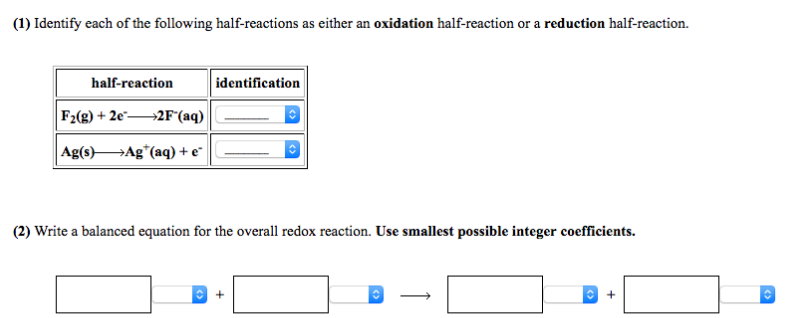# Problem: (1) Identify each of the following half-reactions as either an  oxidation half-reaction or a reduction half-reaction. (2) Write a balanced equation for the overall redox reaction.  Use smallest possible integer coefficients.

###### FREE Expert Solution
82% (9 ratings)###### Problem Details

(1) Identify each of the following half-reactions as either an  oxidation half-reaction or a reduction half-reaction.

(2) Write a balanced equation for the overall redox reaction.  Use smallest possible integer coefficients.